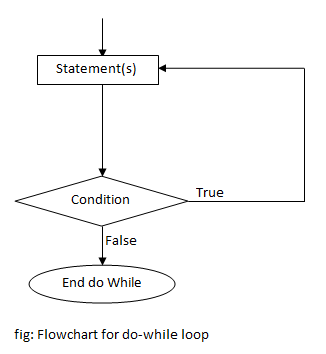# do-while loop in C Programming

Looping is a process of repeating a certain group of statements until a specified condition is satisfied. There are three types of loop in C. They are:

Do-while loop is an exit controlled loop i.e. the condition is checked at the end of loop. It means the statements inside do-while loop are executed at least once even if the condition is false. Do-while loop is an variant of while loop. In order to exit a do-while loop either the condition must be false or we should use break statement.

## Syntax of do-while loop

```do
{
statement(s);
... ... ...
}while (condition);```

## Flowchart of do-while loop## Infinite do-while loop

There may be a condition in a do-while loop which is always true. In such case, the loop will run infinite times. For example,

```do
{
printf("This is infinite loop");
}while(1);```

Any non zero value is considered true in C. To stop an infinite loop, break statement can be used. For example,

```do
{
printf("This loop will run only once");
break;
}while (1);```

## Example of do-while loop

Example: C program to print the table of 5 from 1 to 10.

```#include<stdio.h>
int main()
{
int i=1;
do
{
printf("5 * %d = %dn",i,5*i);
i++;
}while(i<=10);
return 0;
}```

This program prints a multiplication table of 5 from 1 to 10. Do-while loop is used in this program. Initially, the value of i is 1. On each iteration, the value of i is increased by 1 and condition is tested. When the value of i becomes 11,the condition becomes false and loop is terminated.

Output

```5 * 1 = 5
5 * 2 = 10
5 * 3 = 15
5 * 4 = 20
5 * 5 = 25
5 * 6 = 30
5 * 7 = 35
5 * 8 = 40
5 * 9 = 45
5 * 10 = 50```